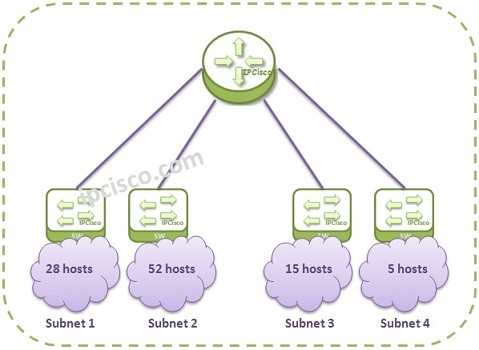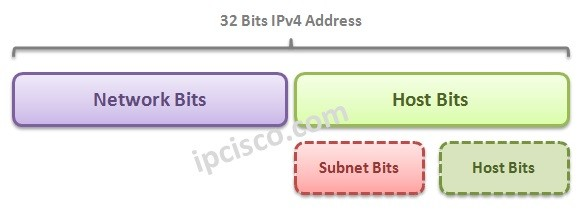## IP Subnetting

As we talked about before, there are two parts in an IP Address. One for them is Network part and the another is Host part. With IP Subnetting, we are adding one more part. This is “Subnet Part”. From the Host part, we borrow some bits and we will use this part for Subnet. In this lesson, we will learn Subnetting with Subnetting Examples.

You can also Practice on Questions Page!As a basic definion, Subnetting is dividing the network into smaller network groups and by doing this, using the IP Address Block more efficient.

For Subnetting, Subnet Masks are used. Subnets masks are 32 bit addresses like IP Addresses. Subnet Masks are used with IP Addresses. The 1s represents the network parts, and 0s represents the host parts.

We can show Subnet Masks with four octets like IP addresses (255.255.255.0) or we can show it like /X . Here, for the 255.255.255.0 Subnet Mask, we can use /24. This means that the first 24 bit is full of 1s and it is network part.

### CIDR versus VLSM

In Subnetting, there are two important terms. These are :

CIDR (Classless Inter Domain Routing)

CIDR (Classless Inter Domain Routing) is the term that is used for using IP addresses independent from their traditional IP Classes. In other words, CIDR is using IP addresses without classes.

VLSM (Variable Lenght Subnet Mask) is the term that is used for using different Subnet Mask for different sun networks. In aother words, it is the mechanism that allows different Subnet Masks and provide division of a network into sub networks. It is like Subnet of subnets.

CIDR is used on the addresses that will advertise to the internet. So, it is used in the Internet Service Provider part.VLSM is used in a company or in smaller networks to use IP address spaces ideally.
Subnetting is one of the important lessons of Networking. So, we will show this with more examples.

### Special Subnets

In Subnetting some Subnet Masks are used specifically sometimes. These are /24, /30, /31/ and /32.

/24 is the Subnet Mask that is usually used in the local networks by default.
/32 is the Subnet Mask used generally on Loopback and System interfaces.
/30 is also widely used in Service Provider Networks for point-to-point connections.

Loopback Interface is the “virtual” interfaces. There can be many Loopback interfaces in a Router. Loopback Interfaces are used for its “always up and never physically down” characteristics generally. We give these Loopback Interfaces a /32 Loopback IP address.

There is also a System Address that is used on Alcatel-Lucent Service Routers. This is a specific loopback address that provide to reach the router’s itself. This address is very important for ALU routers.It is used in many protocol configurations. System addresses are /32 IP addresses.

Now, let’s practice what we have learned with Subnetting Examples.

## Subnetting Examples

In this part, we will see four different Subnetting Examples. With these Subnetting Examples, you will learn this lesson very well.

### IP Subnetting Examples: Example 1

In the first one of the Subnetting Examples, we will use, 192.168.5.85 /24 Address.Let’s determine the network and host part of this address. This is the first example, so we are starting with an easy example.

For this example, firstly we will convert this decimal numbers to the binary equals. As you can see below, the 1s in the Subnet Mask will show the number of bits that network part has. And the 0s will show the host part bits.

Subnet Mask : 11111111. 11111111. 11111111.00000000

So, here, the first 24 bits (First 3 octets) are network bits and the last 8 bits (Last octet) are the host bits.

For this IP and Subnet Mask, to determine the Network Address of this IP address, we will use “AND” operation between IP Address and Subnet Mask in binary mode.

SubM : 11111111. 11111111. 11111111.00000000
AND : 11000000. 10101000.00000101.00000000

When we use AND operation with this binary numbers, as you can see, the last octet will be multiple with zero (AND is Multiplication). So the result of this multiplication will be 192.168.5.0. Here, the first three octets will be same as IP address and the last octet will be full of 0s.

For this example our broadcast address will be 192.168.5.255. AS you can see, all the host bits are full of 1s for broadcast address. The other addresses in the middle through 192.168.5.1 to 192.168.5.254 are host addresses.

### IP Subnetting Examples: Example 2

In the second one of Subnetting Examples, we will do a little more complex example. This time our IP address will be 10.128.240.50/30.

Here, there is a challenge in front of us. As you can see, we have seen the /30 and write 255.255.255.252. How can we do this? Let’s see bit by bit.

/30 means that the subnet mask has 30 bits 1s and 2 bits 0s. Remember the total Subnet Mask is 32 bits. So in binary mode our Subnet Mask is:

11111111.11111111.11111111.11111100 (First 30 bits are 1s and 2 bits are 0s)

And the decimal equal of this Subnet Mask is : 255.255.255.252

Now, let’s determine the network, broadcast and host addresses of this prefix. An IP address with Subnet Mask called Prefix.So, we will write the binary equals of IP address and Subnet and use AND again.

SubM : 11111111.11111111.11111111.11111100
AND : 00001010.10000000.11110000.00110000

The result of AND operation is the Network Address. This is 00001010.10000000.11110000.00110000 in b?nary. The decimal value of this is 10.128.240.48.

Here, the last two bits are host bits and the other bits are network bits. When we set all the host bits with 1s, we will find the Broadcast Address. This is 00001010.10000000.11110000.00110011 in binary. The decimal value is 10.128.240.51.

The middle addresses can be used for hosts. These addresses are 10.128.240.49 and 10.128.240.50.

Host Addresses : 10.128.240.49 and 10.128.240.50

/30 addresses are generally used in Service Provider Networks. So, you can work with /30 too much in the future.

You can also Practice on Questions Page!

### Subnetting Example 3

Now, let’s do a comparison example and see the benefits of Subnetting.